Question

# Assume you wish to evaluate the risk and return behaviors associated with various combinations of two​ stocks, Alpha Sof...

Assume you wish to evaluate the risk and return behaviors associated with various combinations of two​ stocks, Alpha Software and Beta​ Electronics, under three possible degrees of​ correlation: perfect​ positive, uncorrelated, and perfect negative. The average return and standard deviation for each stock appears​ here:

Asset   Average Return,overbar r                   Risk (Standard Deviation), s
Alpha   5.1%   30.3%
Beta                    11.2%      50.5%

a. If the returns of assets Alpha and Beta are perfectly positively correlated​ (correlation coefficient equals plus 1​), over what range would the average return on portfolios of these stocks​ vary? In other​ words, what is the highest and lowest average return that different combinations of these stocks could​ achieve? What is the minimum and maximum standard deviation that portfolios Alpha and Beta could​ achieve?

b. If the returns of assets Alpha and Beta are uncorrelated​ (correlation coefficient equals 0​), over what range would the average return on portfolios of these stocks​ vary? What is the standard deviation of a portfolio that invests​ 75% in Alpha and​ 25% in​ Beta? How does this compare to the standard deviations of Alpha and Beta​ alone?

c. If the returns of assets Alpha and Beta are perfectly negatively correlated​ (correlation coefficient equals negative 1​), over what range would the average return on portfolios of these stocks​ vary? Calculate the standard deviation of a portfolio that invests​ 62.5% in Alpha and​ 37.5% in Beta.

________________________________

a. If the returns of assets Alpha and Beta are perfectly positively correlated​ (correlation coefficient equals plus 1​), the range is between ______​% and ______​% ​(Round to one decimal​ place.)

The range of the standard deviation is between _____​% and _____​% ​(Round to one decimal​ place.)

b. If the returns of assets Alpha and Beta are uncorrelated​ (correlation coefficient equals 0​), the range is between _______​% and ______% ​(Round to one decimal​ place.)

The standard deviation of a portfolio that invests​ 75% in Alpha and​ 25% in Beta is _____​%.​(Round to two decimal​ places.)

c. If the returns of assets Alpha and Beta are perfectly negatively correlated​ (correlation coefficient equals negative 1​), the range is between ______​% and _____​%

​(Round to one decimal​ place.)

The standard deviation of a portfolio that invests​ 62.5% in Alpha and​ 37.5% in Beta is ____​%. ​(Round to two decimal​ places.)

If the returns of assets Alpha and Beta are perfectly positively correlated​ (correlation coefficient equals plus 1​), the range is between 5.1% and 11.2​% ​(Round to one decimal​ place.)

The range of the standard deviation is between 5.1​% and 50.5% ​(Round to one decimal​ place.)

b. If the returns of assets Alpha and Beta are uncorrelated​ (correlation coefficient equals 0​), the range is between 5.1% and 11.2% ​(Round to one decimal​ place.)

The standard deviation of a portfolio that invests​ 75% in Alpha and​ 25% in Beta is 25.9964​%.​(Round to two decimal​ places.)

c. If the returns of assets Alpha and Beta are perfectly negatively correlated​ (correlation coefficient equals negative 1​), the range is between 5.1% and 11.2​%

​(Round to one decimal​ place.)

The standard deviation of a portfolio that invests​ 62.5% in Alpha and​ 37.5% in Beta is 0.08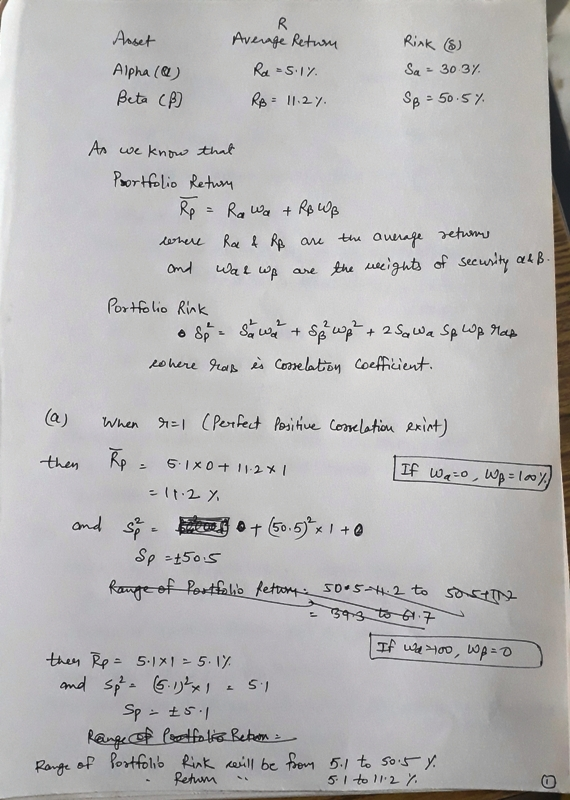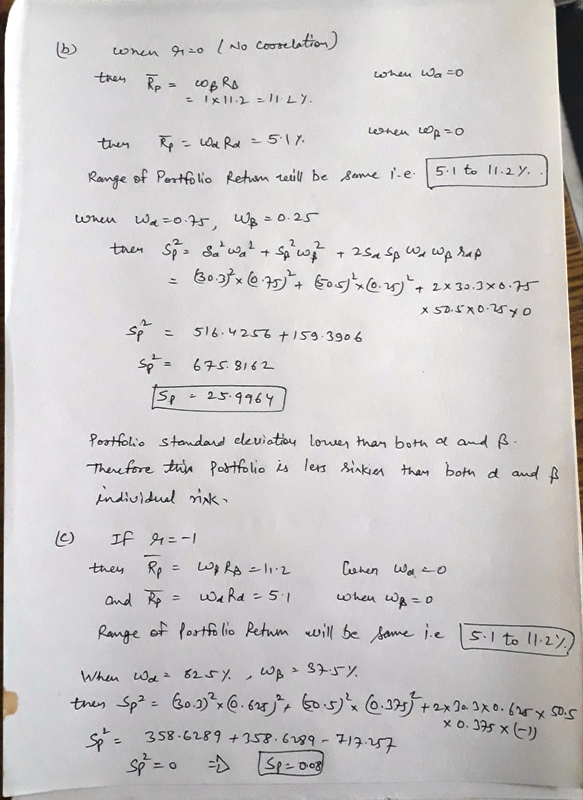​%. ​

#### Earn Coins

Coins can be redeemed for fabulous gifts.

Similar Homework Help Questions
• ### Assume you wish to evaluate the risk and return behaviors associated with various combinations of assets...

Assume you wish to evaluate the risk and return behaviors associated with various combinations of assets V and W under three assumed degrees of​ correlation: perfect​ positive, uncorrelated, and perfect negative. The following average return and risk values were calculated for these​ assets: Asset    Average​ Return, r Risk​ (Standard Deviation), s V 7.9​% 4.6​% W 12.7​% 9.7​% a. If the returns of assets V and W are perfectly positively correlated​ (correlation coefficient = + 1​), describe the range of​...

• ### Correlation, risk, and return Matt Peters wishes to evaluate the risk and return behaviors associated with...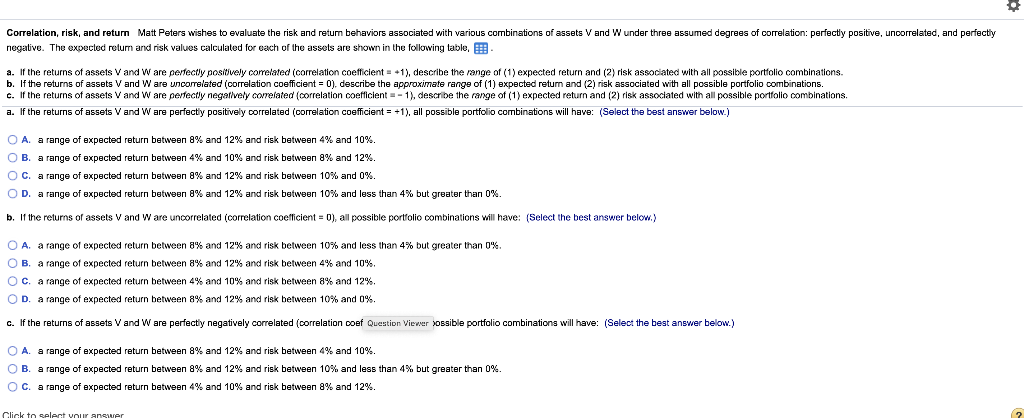Correlation, risk, and return Matt Peters wishes to evaluate the risk and return behaviors associated with various combinations of assets V and W under three assumed degrees of correlation: perfectly positive, uncorrelated, and perfectly negative. The expected return and risk values calculated for each of the assets are shown in the following table, B a. If the returns of assets V and W are perfectly positively correlated correlation coefficient = +1), describe the range of (1) expected return and (2)...

• ### LG4 5-14 Correlation, risk, and return Matt Peters wishes to evaluate the risk and return behaviors...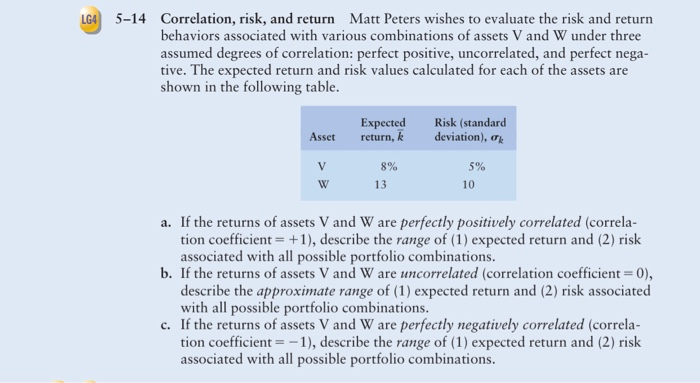LG4 5-14 Correlation, risk, and return Matt Peters wishes to evaluate the risk and return behaviors associated with various combinations of assets V and W under three assumed degrees of correlation: perfect positive, uncorrelated, and perfect nega- tive. The expected return and risk values calculated for each of the assets are shown in the following table. Asset Expected return, k Risk (standard deviation), V 5% 8% 13 a. If the returns of assets V and W are perfectly positively correlated...

• ### 2. 3: Risk and Rates of Return: Risk in Portfolio Context Risk and Rates of Return:...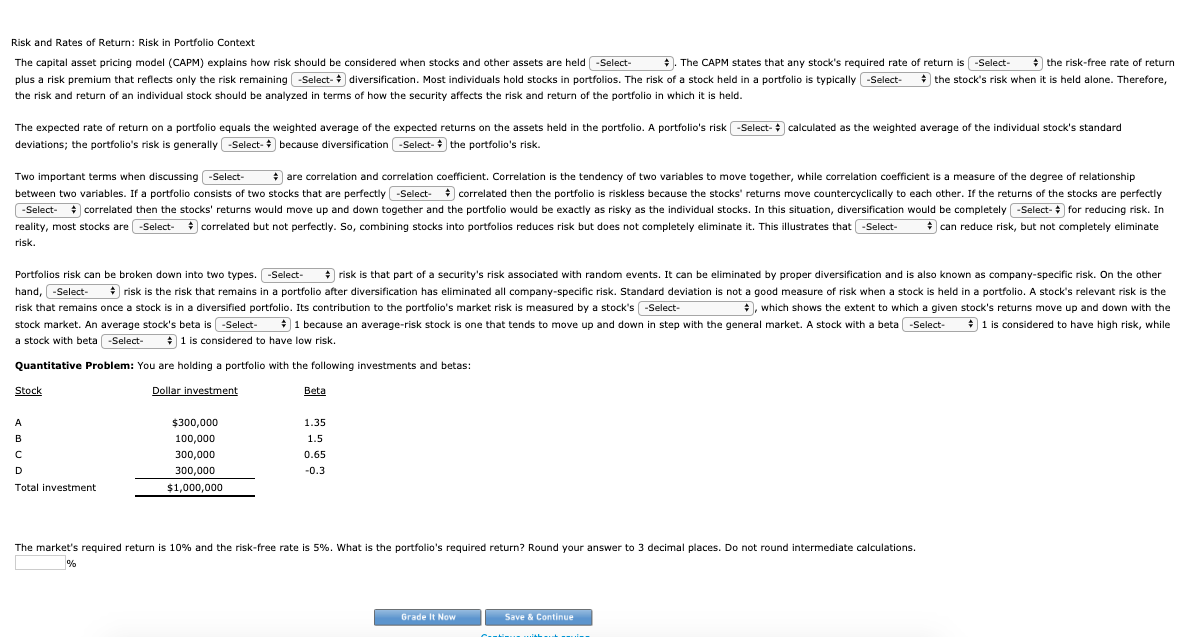2. 3: Risk and Rates of Return: Risk in Portfolio Context Risk and Rates of Return: Risk in Portfolio Context The capital asset pricing model (CAPM) explains how risk should be considered when stocks and other assets are held . The CAPM states that any stock's required rate of return is the risk-free rate of return plus a risk premium that reflects only the risk remaining diversification. Most individuals hold stocks in portfolios. The risk of a stock held in...

• ### P.14 An investor holding a portfolio consisting of two stocks invests 25% of assets in Stock...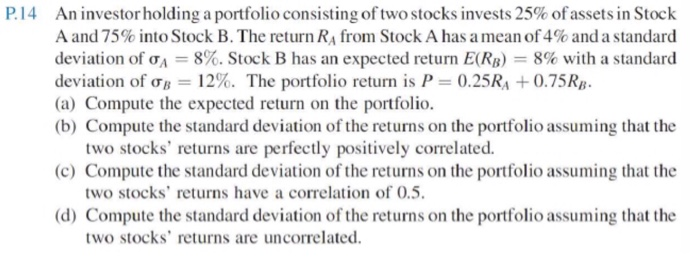P.14 An investor holding a portfolio consisting of two stocks invests 25% of assets in Stock A and 75% into Stock B. The return RA from Stock A has a mean of 4% and a standard deviation of A = 8%. Stock B has an expected return E(RB) = 8% with a standard deviation of ob = 12%. The portfolio return is P = 0.25RA +0.75RB. (a) Compute the expected return on the portfolio. (b) Compute the standard deviation of...

• ### od The capital asset pricing model (CAPM) explains how risk should be considered when stocks and...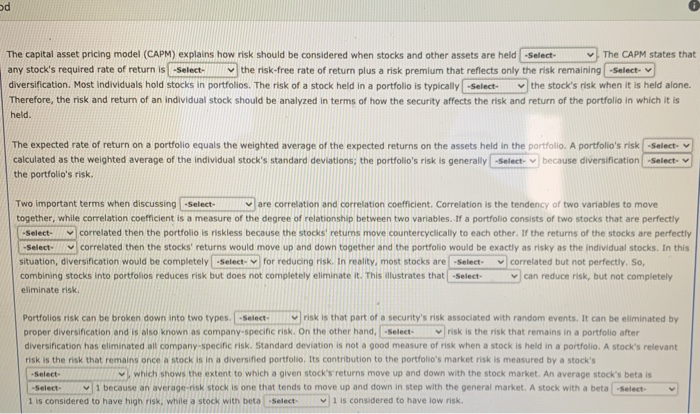od The capital asset pricing model (CAPM) explains how risk should be considered when stocks and other assets are held -Select- The CAPM states that any stock's required rate of return is -Select the risk-free rate of return plus a risk premium that reflects only the risk remaining -Select- diversification. Most individuals hold stocks in portfolios. The risk of a stock held in a portfolio is typically -Select the stock's risk when it is held alone. Therefore, the risk and...

• ### CAPM, portfolio risk, and return Consider the following information for three stocks, Stocks A, B...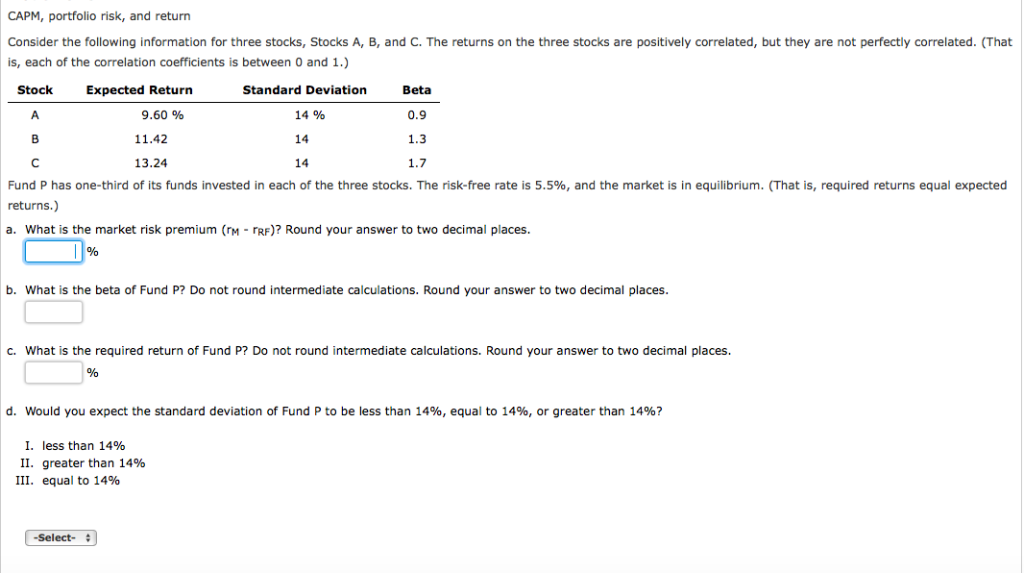CAPM, portfolio risk, and return Consider the following information for three stocks, Stocks A, B, and C. The returns on the three stocks are positively correlated, but they are not perfectly correlated. (That is, each of the correlation coefficients is between 0 and 1.) Stock Standard Deviation 14% 14 14 Beta 0.9 1.3 1.7 Expected Return 9.60 % 11.42 13.24 Fund P has one-third of its funds invested in each of the three stocks. The risk-free rate is 5.5%, and...

• ### CAPM, portfolio risk, and return Consider the following information for three stocks, Stocks A, B, and...

CAPM, portfolio risk, and return Consider the following information for three stocks, Stocks A, B, and C. The returns on the three stocks are positively correlated, but they are not perfectly correlated. (That is, each of the correlation coefficients is between 0 and 1.) Stock Expected Return Standard Deviation Beta A 9.87 % 14 % 0.9 B 11.16 14 1.2 C 12.88 14 1.6 Fund P has one-third of its funds invested in each of the three stocks. The risk-free...

• ### Stocks A and B each have an expected return of 15%, a standard deviation of 17%,...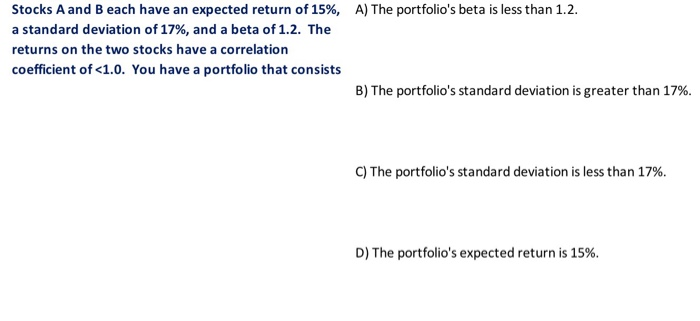Stocks A and B each have an expected return of 15%, a standard deviation of 17%, and a beta of 1.2. The returns on the two stocks have a correlation coefficient of <1.0. You have a portfolio that consists A) The portfolio's beta is less than 12. B) The portfolio's standard deviation is greater than 17%. C) The portfolio's standard deviation is less than 17%. D) The portfolio's expected return is 15%.

• ### CAPM, PORTFOLIO RISK, AND RETURN Consider the following information for stocks A, B, and C. The...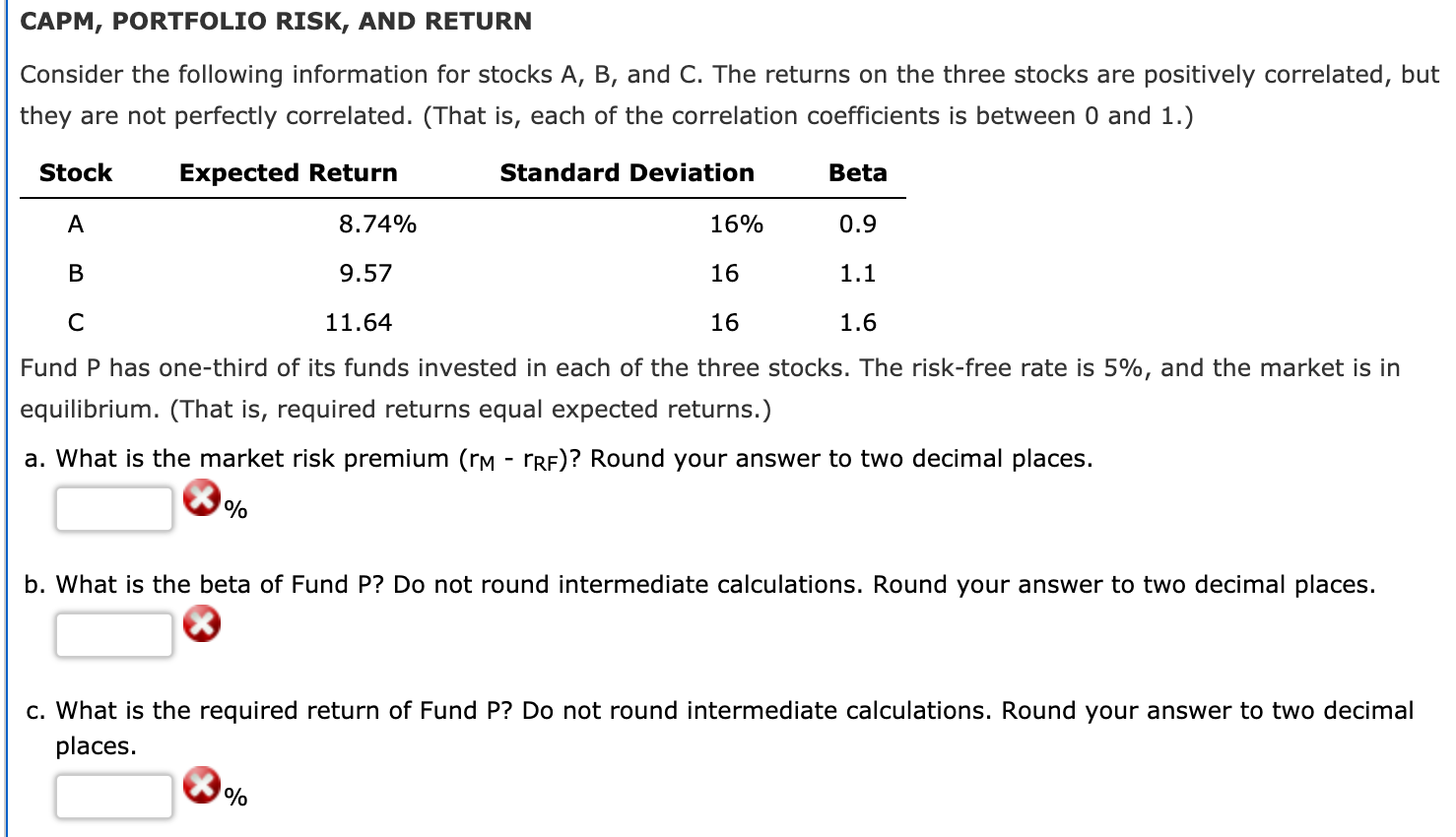CAPM, PORTFOLIO RISK, AND RETURN Consider the following information for stocks A, B, and C. The returns on the three stocks are positively correlated, but they are not perfectly correlated. (That is, each of the correlation coefficients is between 0 and 1.) Stock Expected Return Standard Deviation Beta A 8.74% 16% 0.9 B 9.57 16 1.1 C 11.64 16 1.6 Fund P has one-third of its funds invested in each of the three stocks. The risk-free rate is 5%, and...